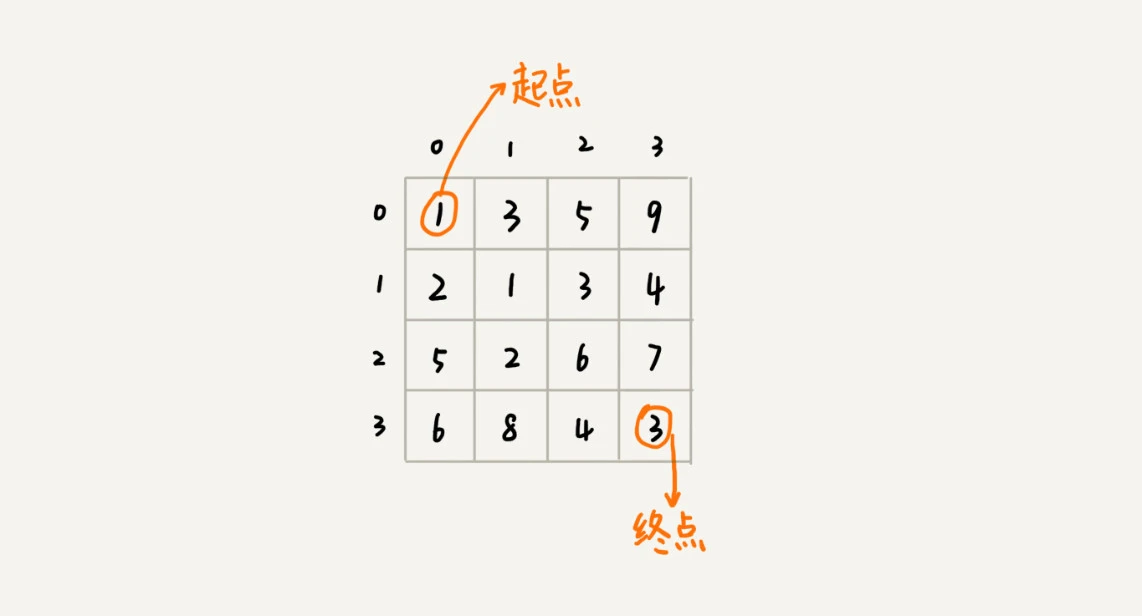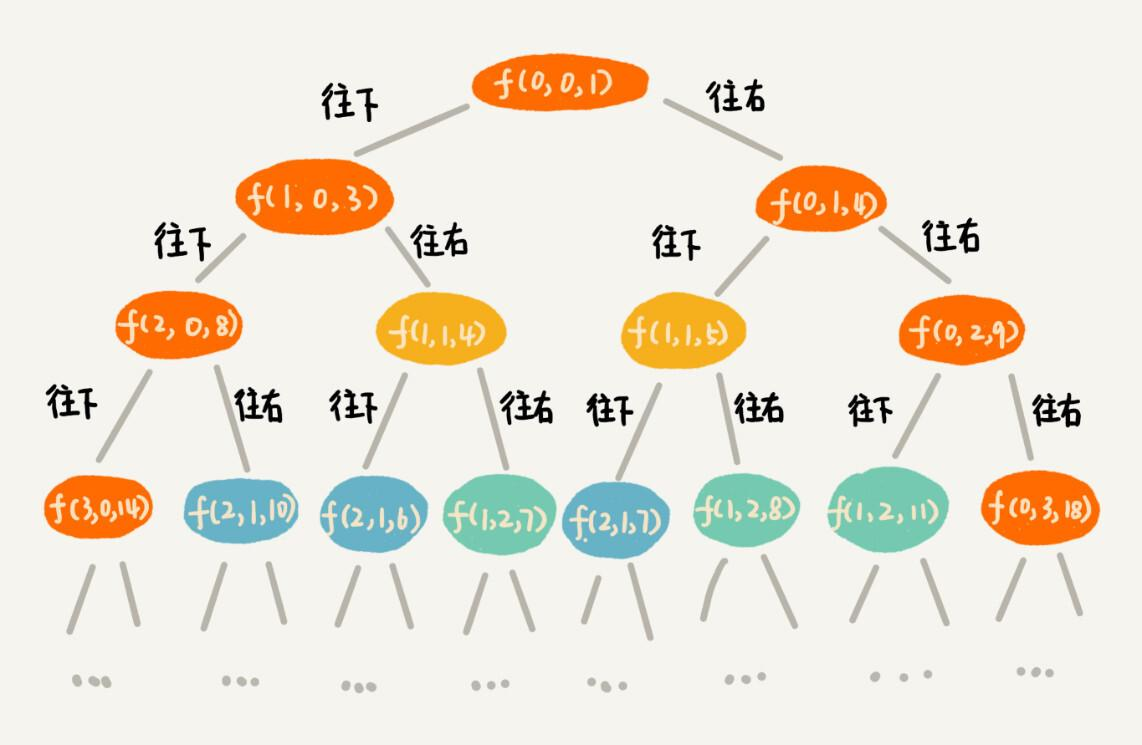# 深入浅出动态规划算法(中)

## 一，“一个模型三个特征”理论讲解

### 4. “一个模型三个特征”实例剖析`min_dist(i, j)` 可以通过 `min_dist(i, j-1)``min_dist(i-1, j)` 两个状态推导出来，所以这个问题符合“最优子结构”。

```cpp min_dist(i, j) = min(min_dist(i-1,j), min_dist(i, j-1))```

## 二，两种动态规划解题思路总结

### 1. 状态转移表法

1，回溯解法的 `C++` 代码如下：

```cpp // leetcode64. 最小路径和. 回溯法-会超出时间限制 class Solution { private: int minDist = 10000; void minDistBT(vector<vector<int>>& grid, int i, int j, int dist, int m, int n) { if (i == 0 && j == 0) dist = grid; if (i == m-1 && j == n-1) { if (dist < minDist) minDist = dist; return; } if (i < m-1) { minDistBT(grid, i + 1, j, dist + grid[i+1][j], m, n); // 向右走 } if (j < n-1) { minDistBT(grid, i, j + 1, dist + grid[i][j+1], m, n); // 向下走 } } public: int minPathSum(vector<vector<int>>& grid) { int m = grid.size(); int n = grid.size(); int dist = 0; minDistBT(grid, 0, 0, dist, m, n); return minDist; } };```2，动态规划解法的 `C++` 代码如下：

```cpp // 对应 leetcode64. 最小路径和 class Solution { // 动态规划：状态转移表法 public: int minPathSum(vector>& grid) { int m = grid.size(); int n = grid.size(); vector > states(m, vector(n, 0)); // 第一个阶段初始化 int sum = 0; for(int i=0; i<n;i++){ // 初始化 states 的第一行数据 sum += grid[i]; states[i] = sum; } sum = 0; for(int j=0; j<m; j++){ // 初始化 states 的第一列数据 sum += grid[j]; states[j] = sum; }

``````    // 分阶段求解，下层状态的值是基于上一层状态来的
for(int i=1; i<m; i++){
for(int j=1; j<n; j++){
states[i][j] = grid[i][j] + std::min(states[i-1][j],states[i][j-1]);
}
}
return states[m-1][n-1];
}
``````

}; ```### 2. 状态转移方程法

```cpp // 状态转移方程 min_dist(i, j) = w[i][j] + min(min_dist(i, j-1), min_dist(i-1, j))```

```cpp // 对应 leetcode64. 最小路径和 class Solution { // 状态转移方程法 private: int minDist(int i, int j, vector >& matrix, vector >& mem) { // 调用minDist(n-1, n-1); if (i == 0 && j == 0) return matrix; if (mem[i][j] > 0) return mem[i][j];

``````    int minUp = 10000;
if (i - 1 >= 0) minUp = minDist(i - 1, j, matrix, mem);
int minLeft = 10000;
if (j - 1 >= 0) minLeft = minDist(i, j - 1, matrix, mem);
int currMinDist = matrix[i][j] + std::min(minUp, minLeft);

mem[i][j] = currMinDist;

return currMinDist;
}
``````

public: int minPathSum(vector>& grid) { int m = grid.size(); int n = grid.size(); vector > mem(m, vector(n, -1));

``````    return minDist(m - 1, n - 1, grid, mem);
}
``````

}; ```「其他文章」